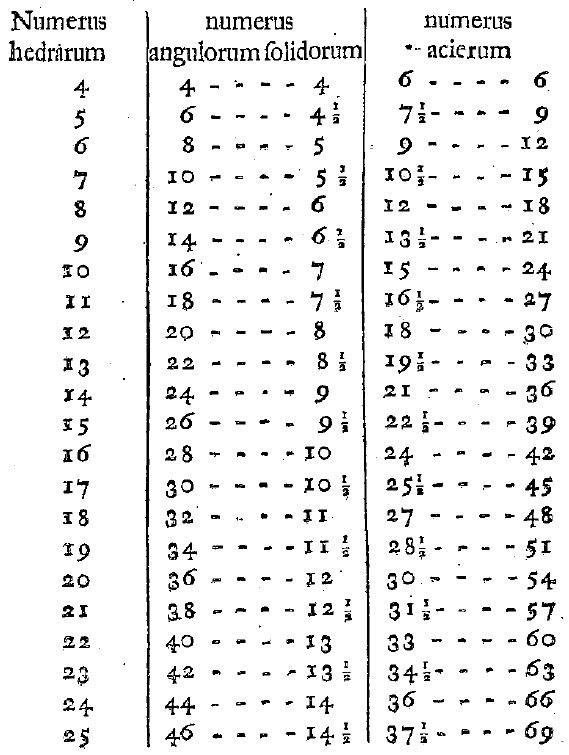# Investigating Euler's Polyhedral Formula Using Original Sources - Additional Results

Author(s):

There are many, many more results in this paper of Euler than can be addressed in this article. We will briefly mention a few additional results.

Proposition 5 (paragraph 37) states:

It is not possible that E + 6 > 3F or E + 6 > 3V.

Proposition 6 (paragraph 42) states:

It is not possible that F + 4 > 2V or V + 4 > 2F.

Euler then combines many of his propositions in an exciting way. From Proposition 2, Corollary 2, we know that 2E ≥ 3F, or equivalently, (3/2)F ≤ E. From Proposition 5, we know that it is not possible that E + 6 > 3F, or equivalently, E ≤ 3F - 6. Therefore, if we know the number of faces, F, of a polyhedron, we now know upper and lower bounds on the number of edges, E:

(3/2)F ≤ E ≤ 3F - 6.

From Proposition 6, we know that F + 4 2V and V + 4 2F, or equivalently, that (1/2)F + 2 ≤ V and V ≤ 2F - 4. Therefore, if we know the number of faces, F, of a polyhedron, we now know upper and lower bounds on the number of vertices, V:

(1/2)F + 2 ≤ V ≤ 2F - 4.

In Proposition 6, Corollary 5 (paragraph 47), Euler uses all of these formulas together and classifies all of the different possible solids by number of faces, vertices, and edges. He produces the following table:Figure 12: Bounds on Vertices and Edges for Given Number of Faces

In the first column are different possible values for the number of faces of a polyhedron ranging from F = 4 to F = 25. For each value of F there is a range of possible values for V and E, and these ranges are the contents of the second and third columns of the table, respectively.

This table can be used in a classroom lesson in a variety of ways. Students can be given the equations above to re-create the table for themselves or simply to extend the table (perhaps for solids with up to 30 faces), or alternatively students could be given the table and asked to locate the equations in Euler's paper that are give rise to these values. Students could be asked to build a similar table: one that gives ranges for the values of F and V given values of E; this is Euler's Corollary 7 to this proposition (paragraph 49); Euler tackles the cases from E = 6 to E = 60.

Propositions 7, 8, and 9 are mentioned in Dr. Sandifer's column in How Euler Did It, and so we will not discuss them here.